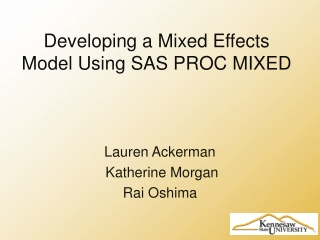DownloadDownload PresentationDeveloping a Mixed Effects Model Using SAS PROC MIXED

# Developing a Mixed Effects Model Using SAS PROC MIXED

Télécharger la présentation## Developing a Mixed Effects Model Using SAS PROC MIXED

- - - - - - - - - - - - - - - - - - - - - - - - - - - E N D - - - - - - - - - - - - - - - - - - - - - - - - - - -
##### Presentation Transcript

1. Developing a Mixed Effects Model Using SAS PROC MIXED Lauren Ackerman Katherine Morgan Rai Oshima

2. Purpose of the Pilot Study • How accurately can participants identify gender from a handwriting sample? 2. Does accuracy improve with feedback?

3. Demographic Information Missing Data!!!

4. Writing Samples 1. 2. 3. 4. 5. 6.

5. Why SAS:PROC SGPANEL • Visualize change over time for each subject

6. PROC SGPLOT proc sgplot data = data_long noautolegend; title 'OLS Trajectories Across Participants'; yaxis min=0 max=50; reg x = time y = score / group = id nomarkers lineattrs = (color = gray pattern = 1 thickness=1); reg x = time y = score / nomarkers lineattrs = (color = red pattern = 1 thickness=3); run; quit;

7. OLS Assumptions • Normality • Homoscedasticity • Zero Correlation

8. Why PROC MIXED?

9. Modeling Covariance Structure Unstructured Covariance Model Independence Covariance Model Compound Symmetry Covariance Model

10. Missing Data PROC REG vs. PROC MIXED MAR Missing At Random

11. proc mixed data = hand_long method=ml; model score = time / solution; run; proc reg data = hand_long; model score = time; run; quit;

12. General Multilevel Model

13. Independence vs. Multilevel Model

14. Unconditional Growth Model PROC MIXED Output PROC REG Output

15. Covariances and Correlations Covariances Correlation Matrix

16. Multilevel Model with Group

17. Fixed Effects Model Analysis 2 2 2 2 2 2

18. Final Model • Model F provided the best deviance statistic • Satisfaction with handwriting and age were the only significant predictors for intercept • No significant predictor for slope besides time

19. Fit Statistics for Covariance Models Deviance Statistics

20. Pilot Study Results • How accurately can participants identify gender from a handwriting sample? Baseline 69.91% accuracy; 95% CI (65.60%,74.23%) Time important predictor (Estimate 1.73, p<0.01) 2. Does accuracy improve with feedback? Group not significant (Estimate 0.37, p = 0.79)

21. Conclusion Why SAS? • Graphical and mixed effects modeling capability Why PROC MIXED? • Allows autocorrelation and homoscedasticity • Flexibility in modeling the within subject variability • Handles missing data • Inclusion of time-varying predictors